Author: Oscar Cronquist Article last updated on September 15, 2021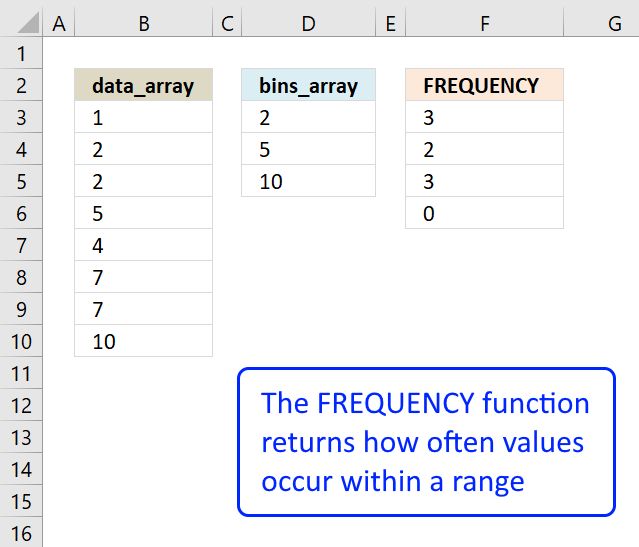The FREQUENCY function calculates how often values occur within a range of values and returns a vertical array of numbers. It returns an array that is one more item larger than the bins_array.

The image above demonstrates the FREQUENCY function cell range F3:F6.

=FREQUENCY(B3:B10, D3:D5)

This formula must be entered as an array formula, however, Excel 365 subscribers may and should enter it as a regular formula. The formula will automatically extend or "spill" values to cells below as far as needed provided the cells are empty.

Microsoft calls these formulas "dynamic arrays" and Excel returns #SPILL! error if cells below are not empty.

Follow these steps to create an array formula if you own an earlier Excel version. I am using the example above, see image above.

### Excel Function Syntax

FREQUENCY(data_array, bins_array)

### Arguments

 data_array An array or cell range for which you want to determine frequencies. bins_array The intervals which you want to group the values in.

### Comment

The FREQUENCY function returns two or more values in a vertical array, blank cells and text strings are ignored. This means that you can only use the function with numerical values.

## 1. FREQUENCY function example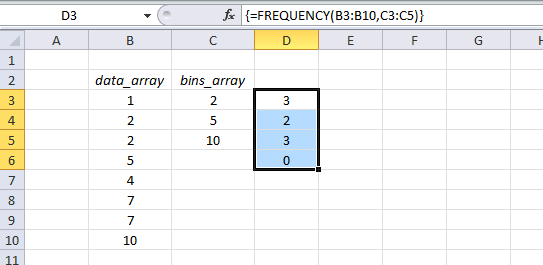(Array) formula in cell range D3:D6:

=FREQUENCY(B3:B10, C3:C5)

### 1.1 How to enter an array formula

1. Select cell range D3:D6
2. Type the formula.
3. Press and hold CTRL + SHIFT keys simultaneously.
4. Press Enter once. Release all keyboard keys.

The formula will now begin and end with a curly bracket, like this: {=array_formula}

They appear automatically, do not enter these characters yourself.

### 1.2 Explaining formula

The first argument is data_array and the second one is bins_array: FREQUENCY(data_arraybins_array)

FREQUENCY(B3:B10, C3:C5)

becomes

FREQUENCY({1; 2; 2; 5; 4; 7; 7; 10}, {2; 5; 10})

and returns a vertical array of numbers: {3230}.

There are 3 values that are smaller or equal to the first value (2) in the bins_array: 1, 2, 2.

There are 2 values that are larger than 2 and smaller or equal to the second value (5) in the bins_array: 4, 5

There are 3 values that are larger than 5 and smaller or equal to the third value (10) in the bins_array: 7, 7, 10

There are 0 (zero) values that are larger than 10 in the bins_array.

The FREQUENCY function returns an array of numbers and it has one more element than the bins_array. In this example the bins_array has 3 values, FREQUENCY function returns 4 values.

## 2. FREQUENCY function with a condition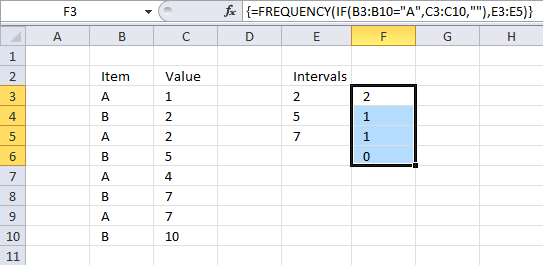The image above shows the FREQUENCY function entered in F3:F6 using an IF function to filter values based on a condition.

Array formula in cell range F3:F6:

=FREQUENCY(IF(B3:B10="A",C3:C10,""),E3:E5)

### 2.1 Explaining the calculation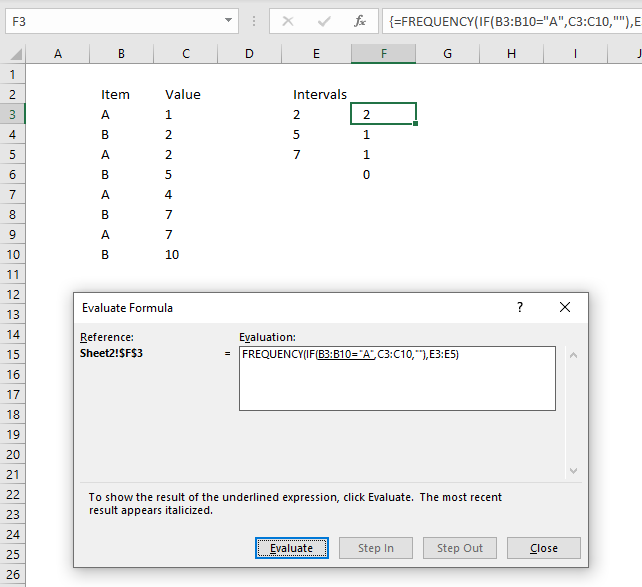I recommend using the "Evaluate Formula" feature to learn more about formulas. This tool allows you to examine formula calculations in detail. It also lets you troubleshoot formulas, what is making my formula return an error?

Go to tab "Formulas" on the ribbon and press with left mouse button on the "Evaluate Formula" button. A dialog box appears, press with left mouse button on the "Evaluate" button to move to the next step in formula calculation. Press with left mouse button on "Close" button to dismiss the dialog box.

#### Step 1 - Filter values

The IF function lets you return a value if a logical expression returns TRUE and another value if FALSE, the logical expression usually returns a boolean value but their equivalents work just fine. FALSE -> 0 (zero), TRUE -> any number.

IF(B3:B10="A",C3:C10,"")

We want to compare the values in cell range B3:B10 with "A" and if they match then return the corresponding value on the same row in cell range C3:C10. IF no match return a blank "".

IF(B3:B10="A",C3:C10,"")

becomes

IF({"A"; "B"; "A"; "B"; "A"; "B"; "A"; "B"}="A",{1; 2; 2; 5; 4; 7; 7; 10},"")

becomes

IF({TRUE; FALSE; TRUE; FALSE; TRUE; FALSE; TRUE; FALSE}, {1; 2; 2; 5; 4; 7; 7; 10},"")

and returns this array: {1; ""; 2; ""; 4; ""; 7; ""}.

#### Step 2 - Frequency

The FREQUENCY function uses the array from the IF function in the first argument, blank values "" are ignored.

FREQUENCY(IF(B3:B10="A",C3:C10,""),E3:E5)

becomes

FREQUENCY({1; ""; 2; ""; 4; ""; 7; ""},E3:E5)

becomes

FREQUENCY({1; ""; 2; ""; 4; ""; 7; ""},{2; 5; 7})

and returns {2; 1; 1;0} in cell range F3:F6.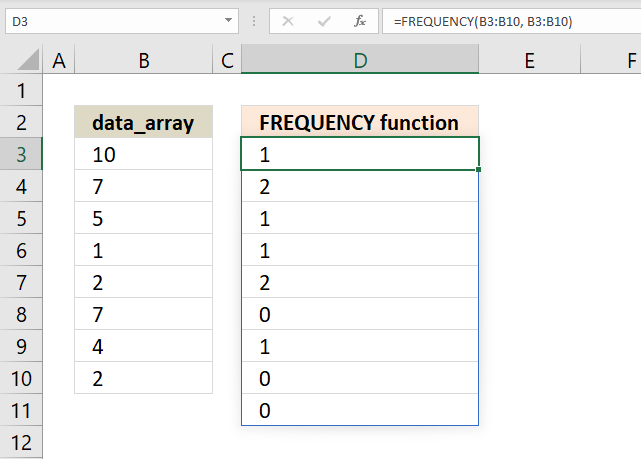The image above shows what the FREQUENCY function returns if you use the same numbers in the data_array and the bins_array.

Formula in cell range D3:D11:

=FREQUENCY(B3:B10, B3:B10)

The FREQUENCY function counts how many values there are for each number for the first instance of a particular number. For example, value 10 exist only once and the function returns 1.

Value 7 exists twice in the list and the corresponding value in the array returns 2, however, only the first instance returns the count. The remaining values for that particular value returns 0 (zero).

This can be used to identify unique distinct numbers in a list among many other things. Check out the FREQUENCY function category to find formulas that contains the FREQUENCY function.

## 3. FREQUENCY function and 3D ranges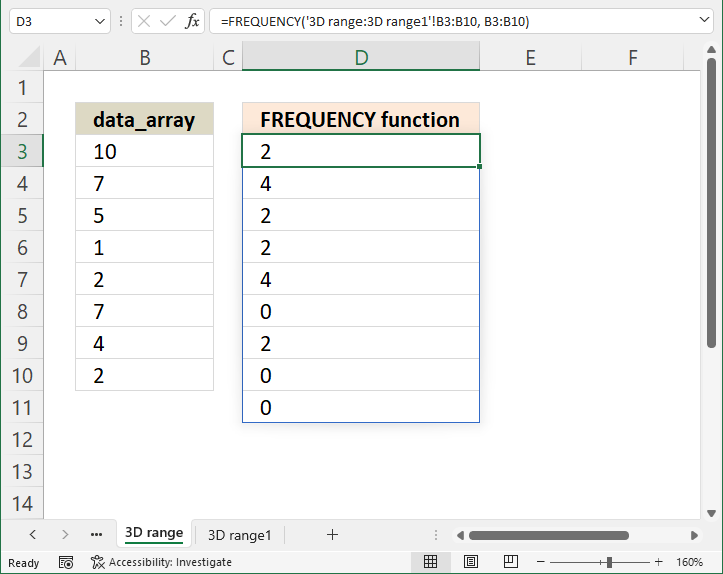This example demonstrates how to use the FREQUENCY function with 3D references. 3D ranges are cell ranges across worksheets meaning a cell reference to a specified number of worksheets in a workbook.

This lets you work with data from multiple worksheets using the FREQUENCY function, I will show you how to build such formula. Other Excel functions that can work with 3D ranges are SUM, AVERAGE, AVERAGEA, COUNT, COUNTA, MAX, MAXA, MINMINAPRODUCTSTDEVSTDEVA, STDEVP, STDEVPA, VAR, VARA, VARP, VARPA functions

Array formula in cell D3:

=FREQUENCY('3D range:3D range1'!B3:B10, B3:B10)

### 3.1 How to enter a formula containing 3D rangesThe formula contains a 3-D reference to a range of worksheet names, make sure you rearrange the worksheets so only worksheets you want to include are being used.

1. Select cell range D3:D10.
2. Press with left mouse button on in the formula bar, the prompt appears.
3. Type =FREQUENCY(
4. Press and hold SHIFT key.
5. Select the last worksheet you want to include in the FREQUENCY function.
6. Release SHIFT key.
7. Select cell range B3:B10 with the mouse.
8. Type , (comma)
9. Select cell range B3:B10 with the mouse.
10. Now enter the formula as an array formula (Excel 365 users can skip this step and simply press Enter).
1. Here are the steps sto enter an array formula.
2. Press and hold CTRL + SHIFT keys simultaneously.
3. Press Enter once.
4. Release all keys.

The formula has now a leading and trailing curly bracket, like this: {=array_formula]

Don't enter these characters yourself, they appear automatically. The image above does not show these characters because I use Excel 365 which uses dynamic array formulas that automatically spill values to cells below.

### 3.2 How to rearrange worksheets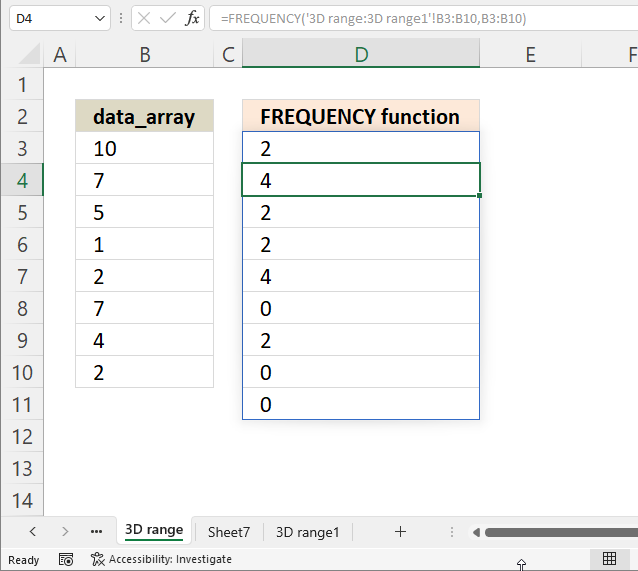The image above shows three worksheet names, "3D range", "Sheet7", and "3D range1". We want to move worksheet "Sheet7" so it is not included in the FREQUENCY function.

1. Press and hold with mouse on worksheet name "Sheet7".
2. Drag with mouse to move the worksheet tab to a new location.
3. Release the mouse button to release the worksheet tab.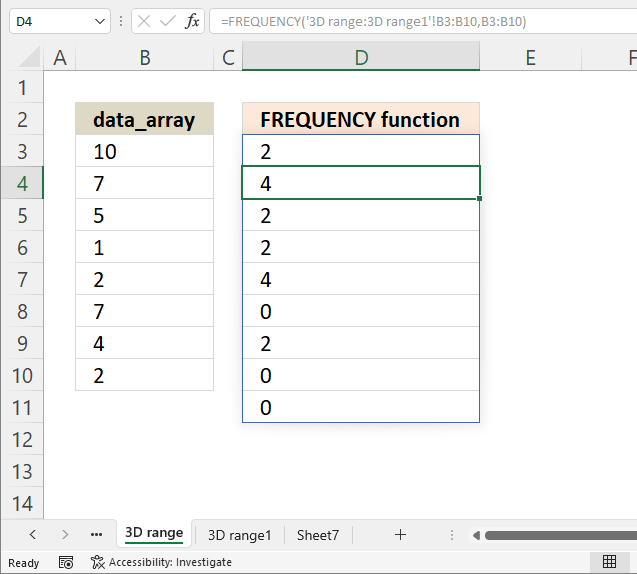The image above shows "Sheet7" in a new location, you can now create a 3D reference to worksheets "3D range" and "3D range1".